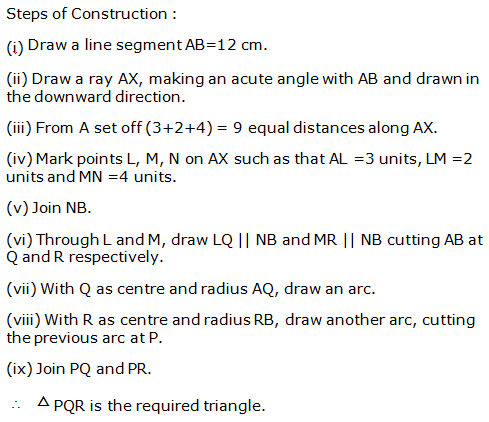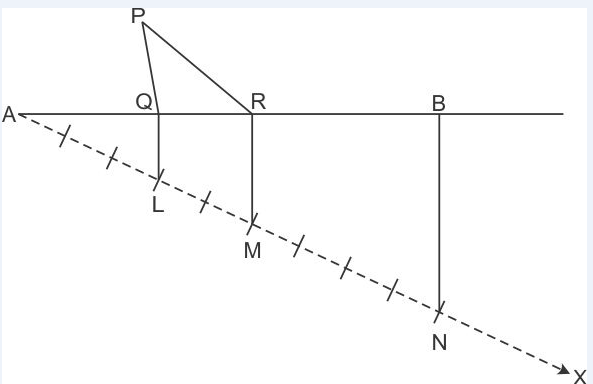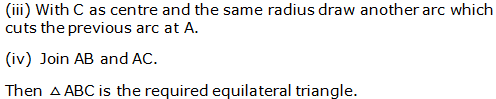# R S AGGARWAL AND V AGGARWAL Solutions for Class 9 Maths Chapter 13 – Geometrical Constructions

## Chapter 13 – Geometrical Constructions Exercise Ex. 13

Question 1

Draw a line segment AB = 5.6 cm
and draw its perpendicular bisector. Measure the length of each part.

Solution 1

Steps
of construction:

1. Draw line segment AB = 5.6 cm
2. With A as centre
and radius more than half of AB, draw two arcs, one on each side of AB.
3. With B as centre
and the same radius as in step 2, draw arcs cutting the arcs drawn in
the previous step at P and Q respectively.
4. Join PQ to intersect AB at M.

Thus,
PQ is the required perpendicular bisector of AB.AM = BM = 2.8 cm

Question 2

Construct a ΔABC
in which BC = 4.5 cm, ∠B = 45° and AB + AC = 8 cm. Justify your construction.

Solution 2

Steps
of construction:

1. Draw BC = 4.5 cm
2. Draw ∠CBX = 45°
3. From ray BX, cut-off line segment BD
equal to AB + AC, i.e. 8 cm.
4. Join CD.
5. Draw the perpendicular bisector of
CD meeting BD at A.
6. Join CA to obtain the required
triangle ABC.Justification:

Clearly, A lies on the
perpendicular bisector of CD.

Now, BD = 8 cm

BA + AD = 8 cm

AB + AC = 8 cm

Hence, ΔABC is the
required triangle.

Question 3

Construct a ΔABC
in which AB = 5.8 cm, ∠B = 60° and BC + CA = 8.4 cm. Justify your construction.

Solution 3

Steps of construction:

1. Draw AB = 5.8 cm
2. Draw ∠ABX = 60°
3. From ray BX, cut off line segment BD
= BC + CA = 8.4 cm.
5. Draw the perpendicular bisector of
6. Join AC to obtain the required
triangle ABC.Justification:

Clearly, C lies on the

CA = CD

Now, BD = 8.4 cm

BC + CD = 8.4
cm

BC + CA = 8.4
cm

Hence,
ΔABC is the
required triangle.

Question 4

Construct a ΔABC
in which BC = 6 cm, ∠B = 30° and AB – AC = 3.5 cm. Justify your construction.

Solution 4

Steps
of construction:

1. Draw base BC = 6 cm
2. Construct ∠CBX = 30°
3. From ray BX, cut off line segment BD
= 3.5 cm (= AB – AC)
4. Join CD.
5. Draw the perpendicular bisector of
CD which cuts BX at A.
6. Join CA to obtain the required
triangle ABC.Justification:

Since A lies on the
perpendicular bisector of CD.

Now, BD = 3.5 cm

cm

AB – AC = 3.5
cm

Hence,
ΔABC is the
required triangle.

Question 5

Construct a ΔABC
in which base AB = 5 cm, ∠A = 30° and AC – BC = 2.5 cm. Justify your construction.

Solution 5

Steps
of construction:

1. Draw base AB = 5 cm
2. Construct ∠BAX = 30°
3. From ray AX, cut off line segment AD
= 2.5 cm (= AC – BC)
4. Join BD.
5. Draw the perpendicular bisector of BD
which cuts AX at C.
6. Join BC to obtain the required
triangle ABC.Justification:

Since C lies on the perpendicular
bisector of BD.

CD = BC

AC – CD = 2.5
cm

AC – BC = 2.5
cm

Hence,
ΔABC is the
required triangle.

Question 6

Construct awhose perimeter is 12 cm and the lengths of whose sides are in the ratio 3:2:4.

Solution 6Question 7

Construct a triangle whose
perimeter is 10.4 cm and the base angles are 45
°
and 120°.

Solution 7

Steps
of Construction:

1. Draw a line segment PQ = 10.4 cm.
2. Construct a 45° angle and bisect it to get NPQ.
3. Construct a 120° angle and bisect it to get MQP.
4. Let
the rays PN and QM intersect at A.
5. Construct
the perpendicular bisectors of PA and QA, to intersect PQ at B and C
respectively.
6. Join
AB and AC.

So, ΔABC is the required triangle.Question 8

Construct a ΔABC
whose perimeter is 11.6 cm and the base angles are 45°
and 60°.

Solution 8

Steps
of Construction:

1. Draw a line segment PQ = 11.6 cm.
2. Construct a 45° angle and bisect it to get NPQ.
3. Construct a 60° angle and bisect it to get MQP.
4. Let
the rays PN and QM intersect at A.
5. Construct
the perpendicular bisectors of PA and QA, to intersect PQ at B and C
respectively.
6. Join
AB and AC.

So, ΔABC is the required triangle.Question 9

In each of the following cases,
given reasons to show that the construction of
ΔABC
is not possible:

AB = 6 cm, ∠A
= 40°
and (BC + AC) = 5.8 cm.

Solution 9

Given,

AB
= 6 cm,
∠A = 40° and (BC + AC)
= 5.8 cm

We
know that the sum of any two sides of a triangle is greater than the third
side.

Here,
we find that BC + AC < AB

Hence,
construction of triangle ABC with given measurements is not possible.

Question 10

In each of the following cases,
given reasons to show that the construction of
ΔABC
is not possible:

AB = 7 cm, ∠A
= 50°
and (BC – AC) = 8 cm.

Solution 10

Given,

AB
= 7 cm,
∠A = 50° and (BC – AC)
= 8 cm

We
know that the sum of any two sides of a triangle is greater than the third
side.

That
is,

AB
+ AC > BC

AB + AC – AC
> BC – AC

AB > BC –
AC

Here,
we find that AB < BC – AC

Hence,
construction of triangle ABC with given measurements is not possible.

Question 11

In each of the following cases,
given reasons to show that the construction of
ΔABC
is not possible:

BC = 5 cm, ∠B
= 80°, ∠C
= 50°
and ∠A
= 60°.

Solution 11

Given,

BC = 5 cm, ∠B
= 80°, ∠C
= 50°
and ∠A
= 60°

We
know that the sum of the measures of three angles of a triangle is 180
°.

Here,
we find that

A + ∠B
+ ∠C = 60° + 80° + 50° = 190° > 180°

Hence,
construction of triangle ABC with given measurements is not possible.

Question 12

In each of the following cases,
given reasons to show that the construction of
ΔABC
is not possible:

AB = 4 cm, BC = 3 cm and AC = 7
cm.

Solution 12

Given,

AB
= 4 cm, BC = 3 cm and AC = 7 cm

We
know that the sum of any two sides of a triangle is greater than the third
side.

Here,
we find that AB + BC = AC

Hence,
construction of triangle ABC with given measurements is not possible.

Question 13

Construct an angle of 67.5°
by using the ruler and compasses.

Solution 13

Steps
for Construction:

1. Draw
a line XY.

2. Take
a point A on XY.

3. With
A as the centre, draw a semi-circle, cutting XY at
P and Q.

4.
Construct
YAC = 90°.

5. Draw
the bisector AB of
XAC. Then YAB = 135°.

6. Draw
the bisector AM of
YAB. Then YAM = 67.5°.Question 14

Construct a square of side 4 cm.

Solution 14

Steps of
Construction:

1. Draw
a line segment PQ = 4 cm.

2.
Construct
QPX = 90° and PQY = 90°.

3. Cut
an arc PS = 4 cm and QR = 4 cm. Join SR.

So, PQRS is the required square.Question 15

Draw an angle of 80°
with the help of a protractor and bisect it. Measure each part of the
bisected angle.

Solution 15

Steps
of construction:

1. Draw ray OB.

2. With the help
of protractor construct an angle AOB of measure 80°.

3. With centre O and convenient radius draw an arc cutting sides
OA and OB at Q and P respectively.

4. With centre Q and radius more than half of PQ, draw an arc.

5. With centre P and the same radius, as in the previous step,
draw another arc intersecting the arc drawn in the previous step at R.

6. Join OR and
produce it to form ray OX.

Then,
OX is the required bisector of
∠AOB.AOX = ∠BOX = 40°

Question 16

Construct a right triangle whose
one side is 3.5 cm and the sum of the other side and the hypotenuse is 5.5
cm.

Solution 16

Steps of construction:

1. Draw BC = 3.5 cm
2. Draw ∠CBX = 90°
3. From ray BX, cut off line segment BD
= AB + AC = 5.5 cm.
4. Join CD.
5. Draw the perpendicular bisector of
CD meeting BD at A.
6. Join AC to obtain the required
triangle ABC.Question 17

Construct a ΔABC
in which ∠B = 45°, ∠C = 60° and the perpendicular from the vertex A to base BC is 4.5
cm.

Solution 17

Steps
of construction:

1. Draw any line XY.
2. Take any point P on XY and draw PQ ⊥ XY.
3. Along PQ, set off PA = 4.5 cm.
4. Through A, draw LM ∥ XY.
5. Construct ∠LAB = 45° and ∠MAC = 60°, meeting XY at B
and C respectively.

Then, ΔABC is the
required triangle.Question 18

Construct an angle ofusing ruler and compasses and bisect it.

Solution 18

Step of Construction:

(i) Draw a line segment OA.

(ii) With O as centre and any suitable radius draw an arc, cutting OA at B.

(iii) With B as centre and the same radius cut the previously drawn arc at C.

(iv) With C as centre and the same radius cut the arc at D.

(v) With C as centre and the radius more than half CD draw an arc.

(vi) With D as centre and the same radius draw another arc which cuts the previous arc at E.

(vii) Join ENow,AOE =900

(viii) Now with B as centre and radius more than half of CB draw an arc.

(iv) With C as centre and same radius draw an arc which cuts the previousat F.

(x) Join OF.

(xi)F is the bisector of rightAOE.Question 19

Construct each of the following
angles, using ruler and compasses:

75°

Solution 19

Steps
of construction:

1. Draw a line segment PQ.
2. With centre P and any
radius, draw an arc which intersects PQ at R.
3. With centre R and same
radius, draw an arc which intersects previous arc at S.
4. With centre S and same
radius, draw an arc which intersects arc in step 2 at T.
5. With centres T and S
and radius more than half of TS, draw arcs intersecting each other at U.
6. Join PU which intersects arc in step 2 at V.
7. With centres V and S
and radius more than half of VS, draw arcs intersecting each other at W.
8. Join PW.

WPQ = 75°Question 20

Construct each of the following
angles, using ruler and compasses:

37.5°

Solution 20

Steps
of construction:

1. Draw a line segment PQ.
2. With centre
P and any radius, draw an arc which intersects PQ at R.
3. With centre
R and same radius, draw an arc which intersects previous arc at S.
4. With centre
S and same radius, draw an arc which intersects arc in step 2 at T.
5. With centres
T and S and radius more than half of TS, draw arcs intersecting each
other at U.
6. Join PU which intersects arc in step
2 at V.
7. With centres
V and S and radius more than half of VS, draw arcs intersecting each other
at W.
8. Join PW. ∠WPQ = 75°
9. Bisect ∠WPQ.

Then,
∠ZPQ = 37.5°Question 21

Construct each of the following
angles, using ruler and compasses:

135°

Solution 21

Steps
of construction:

1. Draw a line segment AB and produce
BA to point C.
2. With centre
A and any radius, draw an arc which intersects
AC at D and AB at E.
3. With centres
D and E and radius more than half of DE, draw two arcs which intersect
each other at F.
4. Join FA which intersects the arc in
step 2 at G.
5. With centres
G and D and radius more than half of GD, draw two arcs which intersect
each other at H.
6. Join HA.

Then,
∠HAB = 135°Question 22

Construct each of the following
angles, using ruler and compasses:

105°

Solution 22

Steps
of construction:

1. Draw a line segment PQ.
2. With centre P and any
radius, draw an arc which intersects PQ at R.
3. With centre R and same
radius, draw an arc which intersects previous arc at S.
4. With centre S and same
radius, draw an arc which intersects arc in step 2 at T.
5. With centres T and S
and radius more than half of TS, draw arcs intersecting each other at U.
6. Join PU which intersects arc in step 2 at V.
7. Now taking T and V as centres, draw arcs with radius more than half the
length TV.
8. Let these arcs intersect
each other at W.
9. Join PW, which is the
required ray making 105°with the given ray PQ.

Then, ∠WPQ = 105°Question 23

Construct each of the following
angles, using ruler and compasses:

22.5°

Solution 23

Steps
of construction:

1. Draw a line segment AB.
2. With centre A and any radius, draw an arc which intersects AB at
C.
3. With centre C and same
radius, draw an arc which intersects previous arc at D.
4. With centre D and same
radius, draw an arc which intersects arc in step 2 at E.
5. With centres E and D
and radius more than half of ED, draw arcs intersecting each other at F.
6. Join AF which intersects arc in step 2 at G.
7. Now taking G and C as centres, draw arcs with radius more than half the
length GC.
8. Let these arcs
intersect each other at H.
9. Join AH which
intersect the arc n step 2 at I.
10. With centres I and C and radius more than half of IC,
draw arcs intersecting each other at J.
11. Join AJ.

Then, ∠JAB = 22.5°Question 24

Construct a Δ
ABC in which BC = 5 cm, AB = 3.8 cm and AC = 2.6 cm. Bisect the largest angle
of this triangle.

Solution 24

Steps
of construction:

1. Draw line segment AC = 2.6 cm.
2. With A as centre and
radius 3.8 cm, draw an arc.
3. With C as centre and
radius 5 cm, draw arc to intersect the previous arc at B.
4. Join AB and BC.

Thus,
ΔABC is the required
triangle.

Largest
side = BC = 5 cm

Largest angle
= ∠A

Steps
of construction:

1. With A as centre and
any radius, draw an arc, which intersect AB at P and AC at Q.
2. With P as centre and
radius more than half of PQ, draw an arc.
3. With Q as centre and
the same radius, draw an arac to intersect the
previous arc at R.
4. Join AR and extend it.

Thus,
∠A is bisected
by ray AR.Question 25

Construct a ΔABC
in which BC = 4.8 cm, ∠B = 45° and ∠C = 75°. Measure ∠A.

Solution 25

Steps
of construction:

1. Draw a line segment BC = 4.8 cm.
2. With centre
B and any radius, draw an arc which intersects BC at P.
3. With centre
P and same radius, draw an arc which intersects previous arc at Q.
4. With centre
Q and same radius, draw an arc which intersects arc in step 2 at R.
5. With centres
R and Q and radius more than half of RQ, draw arcs intersecting each
other at S.
6. Join BS which intersects arc in step
2 at G. Then,
∠SBC = 90°
7. With centre
P and radius more than half of PG, draw an arc.
8. With centre
G and same radius, draw an arc which intersects previous arc at X.
9. Join B and extend it. Then ∠B = 45°
10. Construct ∠TCB = 90° following the steps
given above.
11. With centres
M and H and radius more than half of MH, draw arcs intersecting each
other at Y.
12. Join CY and extend it. Then, ∠C = 75°
13. Extended BX and CY intersect at A.

Thus,
ΔABC is the
required triangle.

m∠A = 60°Question 26

Construct an equilateral trianagle each of whose sides measures 5cm.

Solution 26

Step of construction:

(i) Draw a line segment BC=5cm.

(ii) With B as centre and radius equal to BC draw an arc.Question 27

Construct an equilateral triangle
each of whose altitudes measures 5.4 cm. Measure each of its sides.

Solution 27

Steps
of construction:

1. Draw a line XY.
2. Mark any point P on it.
3. From P, draw PQ ⊥ XY.
4. From P, set off PA = 5.4 cm, cutting PQ at A.
5. Construct ∠PAB = 30°
and PAC = 30°, meeting XY at B
and C respectively.

Then,
ABC is the required equilateral triangle.Question 28

Construct a right-angled triangle
whose hypotenuse measures 5 cm and the length of one whose sides containing
the right angle measures 4.5 cm.

Solution 28

Steps
of construction:

1. Draw a line segment BC = 5 cm.
2. Find the midpoint O of BC.
3. With O as centre
and radius OB, draw a semicircle on BC.
4. With B as centre
and radius equal to 4.5 cm, draw an arc, cutting the semicircle at A.
5. Join AB and AC.

Then,
ΔABC is the
required triangle.error: Content is protected !!